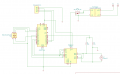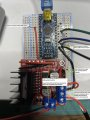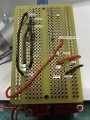# Unable to get DC motor to run for project

#### blaravie

Joined May 25, 2021
11
Hello all,

I am at a loss for what I am doing wrong in my attempt to run a dc motor with a rotary encoder, l298N, and Arduino Nano.

Here is the code I am running:
Code:
/* Turbofan Driver
This program used an L298N motor driver with a 12VDC motor.
The 20x4 LCD displays motor speed, turbofan speed, and motor voltage.
An encoder is used to control the motor speed.
Press the button to set speed back to zero.
Hold the button for 2 seconds to set the Maximum Fan RPM
Hold the button for 5 seconds to reset the Maximum Fan RPM to uncapped.
Maximum RPM set is remembered inside the program even after power down.
The system uses to following components:
Arduino Nano
L298N Motor Driver
RS-555SH 12VDC Motor (or equivalent)
2004D I2C LCD Screen
20 PPR Rotary Encoder
12VDC Power Supply
1x 10k resistor
1x 100k resistor
Written and designed by Adam B Johnson
15 January 2021
*/

#define gearRatio 2.27              //fixed gear ratio

#include <Encoder.h>                //Might need to download this library. Tools > Manage Libraries > Search for 'Encoder'
#include <LiquidCrystal_I2C.h>      //Might also need to download this one in the same way.
#include <EEPROM.h>                 //This should have been included when you installed Arduino IDE. No downloade needed

//##################  Adjust these values accordingly #######################################

#define chB 2                           //***pin B of the rotary encoder (channel B)
#define chA 3                           //***pin A of the rotary encoder (channel A)
#define motorDir1 9                     //***motor direction forward pin to driver
#define motorDir2 10                    //***motor direction reverse pin to driver
#define motorPWM 6                      //***PWM pin to motor controller
#define pushButton 7                    //***Button pin
#define voltPin A0                      //***Analog pin for motor voltage measurement
#define arduinoVoltage 4.98             //***Actual measured 5 volt pin from the arduino
#define R1 100500.0                     //***Actual resistance of 100k resistor
#define R2 9930.0                       //***Actual resistance of 10k resistor
float rpmPerVolt = (4825 / 12);         //***DC motor max RPM (4825) divided by max voltage (12VDC. Measure both paraments and adjust
LiquidCrystal_I2C lcd(0x3F, 20, 4);     //***Adjust screen address if needed. Use File > Examples > Wire > i2c_scanner to acquire address and change 0x3F as needed.

//##################  End of adjustable parameters #######################################

int pwmMax;
int pwmVal = 0;
bool setFlag = false;

int fanSpeed = 0;                   //speed of the turbofan in RPM
int mtrSpeed = 0;                   //speed of the motor in RPM
int deadBand = 20;                  //minimum PWM signal for the motor to move
float vout = 0.0;                   //Voltage out of the motor
float roughVoltage = 0.0;
int avgInst = 350;                  //this should equal the sizeof(tempArray)
int voltCount = 0;
float tempArray;               //temp array for averaging voltage readings: array size = number of averaging points
float finalVolt = 0.0;              //averaged result
float oldVolt = 0.0;                //check to see if voltage changes after each loop

long timeNew = 0;                   //time stamp at beginning of loop
long timeOld = 0;                   //time stamp at end of end
long tempTimer = 0;
long oldPos = 0;                    //old position of the encoder
long newPos = 0;                    //new position of the encoder

Encoder myEncoder(chA, chB);

void setup() {
//Serial.begin(115200);
pinMode(voltPin, INPUT);
pinMode(motorDir1, OUTPUT);
pinMode(motorDir2, OUTPUT);
pinMode(motorPWM, OUTPUT);
pinMode(pushButton, INPUT_PULLUP);
pinMode(chA, INPUT_PULLUP);
pinMode(chB, INPUT_PULLUP);

myEncoder.write(oldPos);
digitalWrite(motorDir1, HIGH);  //motor direction (FWD = HIGH). DON'T CHANGE. SWITCH THE WIRES IF DIRECTION IS WRONG
digitalWrite(motorDir2, LOW);   //motor direction (REV = LOW). DON'T CHANGE. SWITCH THE WIRES IF DIRECTION IS WRONG
analogWrite(motorPWM, 0);

lcd.init();
lcd.setCursor ( 3, 0 );
lcd.print("Turbofan Driver");
lcd.setCursor ( 0, 2 );
lcd.setCursor( 0, 3);
lcd.setCursor ( 16, 3 );
lcd.print("2021");

delay(4500);
lcd.clear();
screenSetup();
zeroNums();
printSpeeds();
printVoltage();
myEncoder.write(0);
analogWrite(motorPWM, 0);
}

void loop() {
timeNew = millis();

if (voltCount < avgInst) {
vout = (analogRead(voltPin) * arduinoVoltage) / 1024.0;             //Measure your 5VDC rail to ground with your DMM. Change 4.98 to whatever yours is
roughVoltage = vout / (R2 / (R1 + R2));
tempArray[voltCount] = roughVoltage;
} else {
voltCount = 0;
}

if (newPos > 0) {
//Serial.println(newPos);
if (timeNew - timeOld > 300) {
finalVolt = 0.0;
for (int i = 0; i < avgInst; i++) {
finalVolt += tempArray[i];
}
timeOld = timeNew;
finalVolt = finalVolt / avgInst;
if (abs(finalVolt - oldVolt) > 0.03) {
mtrSpeed = rpmPerVolt * finalVolt;
fanSpeed = mtrSpeed / gearRatio;
oldVolt = finalVolt;
clearNums();
printVoltage();
printSpeeds();
}
}
}
voltCount++;

if ((newPos != oldPos) && (newPos > 0) && (newPos < pwmMax - deadBand)) {
analogWrite(motorPWM, pwmVal);
oldPos = newPos;
}
if ((newPos != oldPos) && (newPos <= 0) && (newPos < pwmMax - deadBand)) {
delay(250);
myEncoder.write(0);
analogWrite(motorPWM, 0);
clearNums();
zeroNums();
delay(50);
myEncoder.write(0);
analogWrite(motorPWM, 0);
}
if ((newPos != oldPos) && (newPos > 0) && (newPos >= pwmMax - deadBand)) {
analogWrite(motorPWM, pwmVal);
pwmVal = pwmMax;
mtrSpeed = mtrSpeed = rpmPerVolt * finalVolt;
fanSpeed = mtrSpeed / gearRatio;
}

//Serial.print("buttonPressed");
delay(25);
//Serial.print("buttonStillPressed");
setFlag = false;
delay(500);
for (int i = 0; i < 20; i++) {
lcd.setCursor(i, 0);
lcd.print(" ");
}
lcd.setCursor(1, 0);
lcd.print("Max Speed Set: ");
lcd.setCursor(16, 0);
lcd.print(fanSpeed);
delay(3000);
for (int i = 0; i < 20; i++) {
lcd.setCursor(i, 0);
lcd.print(" ");
}
lcd.setCursor(2, 0);
lcd.print("Max Speed Reset");
delay(3000);
}
for (int i = 0; i < 20; i++) {
lcd.setCursor(i, 0);
lcd.print(" ");
}
lcd.setCursor(3, 0);
lcd.print("Turbofan Stats");
setFlag = true;
}
if (setFlag == false) {
myEncoder.write(0);
analogWrite(motorPWM, 0);
clearNums();
zeroNums();
setFlag = true;
}
}
}

}

void printVoltage() {
if ((finalVolt > 0) && (finalVolt < 10.0)) {
lcd.setCursor (12, 3 );
} else if ((finalVolt < 15.0) && (finalVolt >= 10.0)) {
lcd.setCursor (11, 3 );
} else {
lcd.setCursor (12, 3 );
finalVolt = 0;
}
lcd.print(finalVolt, 2);
}

void printSpeeds() {
if (fanSpeed < 100 && fanSpeed >= 10) {
lcd.setCursor(14, 1);
} else if (fanSpeed < 1000 && fanSpeed >= 100) {
lcd.setCursor (13, 1 );
} else if (fanSpeed < 10000 && fanSpeed >= 1000) {
lcd.setCursor (12, 1 );
} else if (fanSpeed == 0) {
lcd.setCursor (15, 1 );
} else {
lcd.setCursor (15, 1 );
fanSpeed = 0;
}
lcd.print(fanSpeed);
if (mtrSpeed < 100 && mtrSpeed >= 10) {
lcd.setCursor(14, 2);
} else if (mtrSpeed < 1000 && mtrSpeed >= 100) {
lcd.setCursor (13, 2 );
} else if (mtrSpeed < 10000 && mtrSpeed >= 1000) {
lcd.setCursor (12, 2 );
} else if (mtrSpeed == 0) {
lcd.setCursor (15, 2 );
} else {
lcd.setCursor (15, 2 );
mtrSpeed = 0;
}
lcd.print(mtrSpeed);
}

void clearNums() {
for (int i = 11; i < 17; i++) {
for (int j = 1; j < 4; j++) {
lcd.setCursor (i, j);
lcd.print(" ");
}
}
}

void zeroNums() {
oldPos = 0;
mtrSpeed = 0;
fanSpeed = 0;
finalVolt = 0;
delay(100);
printSpeeds();
printVoltage();
}

void screenSetup() {
lcd.setCursor ( 3, 0 );
lcd.print("Turbofan Stats");
lcd.setCursor ( 0, 1 );
lcd.print("Fan Speed:");
lcd.setCursor (17, 1 );
lcd.print("RPM");
lcd.setCursor ( 0, 2 );
lcd.print("Mtr Speed:");
lcd.setCursor (17, 2 );
lcd.print("RPM");
lcd.setCursor ( 0, 3 );
lcd.print("Voltage:");
lcd.setCursor (17, 3 );
lcd.print("VDC");
}
I'm using this schematic:and here is the pictures with the actual arduino/module driver/encoder setup:The DC motor I'm using is this:
https://www.parts-express.com/Mabuchi-Type-Motor-RS-555SH-12V-DC-Motor-9-15V-57mm-x-36mm-125-566

Encoder:
https://datasheet.lcsc.com/szlcsc/ALPS-Electric-EC11E18244AU_C202365.pdf

So I've checked all my continuity and voltage and everything looks good, but I can't get the DC motor to run via the rotary encoder no matter what I do. The encoder works fine with the arduino (by using the example code), same with the screen. I've verified the dc motor works by using a different L298N as well.

Can someone please help me here? It's driving me absolutely crazy that I can't get this to work and I'm out of options. Maybe something wrong with the way I have everything connected? Bad L298N driver? Bad code?

#### ronsimpson

Joined Oct 7, 2019
1,657
L298 pins 3 & 13 are shorted to ground in the schematic.

#### blaravie

Joined May 25, 2021
11
L298 pins 3 & 13 are shorted to ground in the schematic.
Is that wrong?
Someone told me that I should have Out1/Out4 connected to R1 (correctly shown in the schematic) but R2 should be grounded and not connected to Out2/Out3.
Is that right?

Joined Feb 20, 2016
3,939
Take the gnd off the motor as @ronsimpson noted.
Neither side of the motor should be grounded as the L298 needs to determine that.
but R2 should be grounded and not connected to Out2/Out3.
Is that right?
No!

See if the fixes your problems.

#### blaravie

Joined May 25, 2021
11
Take the gnd off the motor as @ronsimpson noted.
Neither side of the motor should be grounded as the L298 needs to determine that.

No!

See if the fixes your problems.
Okay so leave everything like I have it in the picture but remove the ground from R2?

Joined Feb 20, 2016
3,939
yes. There is no gnd (or power) directly connected to the motor. Only via the H bridge.
And you may find the H bridge is now dead because of the gnd on the output.

•Delta Prime

#### blaravie

Joined May 25, 2021
11
Thank you. I will try that and see if it works.

#### blaravie

Joined May 25, 2021
11
So I removed the ground. Now I have OUT1/OUT4 going to R1, OUT2/OUT3 going to R2 and no ground. Still nothing. I will try replacing the L298 and see if it works.

#### blaravie

Joined May 25, 2021
11
So after I said that, I tried this code and it worked great!

It's nice to see it FINALLY moving but now I am as confused as ever. This shows the L298 works, it's getting proper voltage, and the arduino is properly communicating with it. Is there a problem with the code I am using??

Code:
[code]
/**
* Bruno Santos, 2013
* feiticeir0@whatgeek.com.pt
* Small code to test DC motors - 2x with a L298 Dual H-Bridge Motor Driver
* Free to share
**/
//Testing the DC Motors
//Define Pins
//Motor A
int enableA = 6;
int pinA1 = 10;
int pinA2 = 9;
//Motor B
int enableB = 6;
int pinB1 = 9;
int pinB2 = 10;
void setup() {
Serial.begin (9600);
//configure pin modes
pinMode (enableA, OUTPUT);
pinMode (pinA1, OUTPUT);
pinMode (pinA2, OUTPUT);
pinMode (enableB, OUTPUT);
pinMode (pinB1, OUTPUT);
pinMode (pinB2, OUTPUT);
}
void loop() {
//enabling motor A
Serial.println ("Enabling Motor A");
digitalWrite (enableA, HIGH);
//do something
//forward
Serial.println ("Forward");
digitalWrite (pinA1, HIGH);
digitalWrite (pinA2, LOW);
//5s forward
delay (5000);
//reverse
digitalWrite (pinA1,LOW);
digitalWrite (pinA2,HIGH);
//5s backwards
delay (5000);
//stop
digitalWrite (enableA, LOW);
delay (5000);
//enabling motor B
//Since motor B is mounted reversed, PINs must be exchanged
Serial.println ("Enabling Motor A");
digitalWrite (enableB, HIGH);
//do something
//forward
Serial.println ("Forward");
digitalWrite (pinB1, LOW);
digitalWrite (pinB2, HIGH);
//5s forward
delay (5000);
//reverse
digitalWrite (pinB1,HIGH);
digitalWrite (pinB2,LOW);
//5s backwards
delay (5000);
//stop
digitalWrite (enableB, LOW);
delay (5000);
}
[/CODE]

Joined Feb 20, 2016
3,939
There are problems with your code I think.
For one thing, the motorDIRs do not change outside setup as far as I can see so the motor will only run in one direction.
And I cannot figure out the pwm setting for speed control.
Code is not my strong point, sorry.